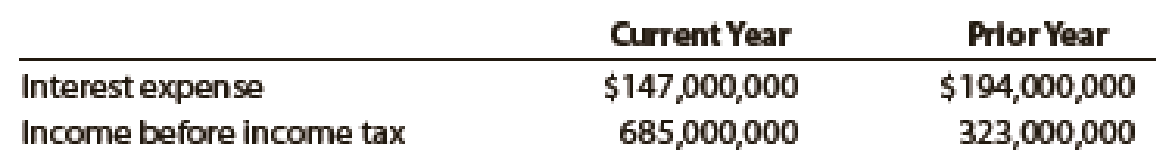# The following data were taken from recent annual reports of Southwest Airlines, which operates a low-fare airline service to more than 50 cities in the United States: a. Determine the times interest earned ratio for the current and preceding years. Round to one decimal place. b. What conclusions can you draw?### Financial Accounting

15th Edition
Carl Warren + 2 others
Publisher: Cengage Learning
ISBN: 9781337272124

#### Solutions

Chapter
Section### Financial Accounting

15th Edition
Carl Warren + 2 others
Publisher: Cengage Learning
ISBN: 9781337272124
Chapter 14, Problem 14E
Textbook Problem
1 views

## The following data were taken from recent annual reports of Southwest Airlines, which operates a low-fare airline service to more than 50 cities in the United States:a. Determine the times interest earned ratio for the current and preceding years. Round to one decimal place. b. What conclusions can you draw?

(a)

To determine

Calculate times-interest-earned ratio for the current year and the prior year.

### Explanation of Solution

Times-Interest-Earned ratio: It is the ratio that quantifies a business ability to pay interest expense. It is calculated as shown below:

Times-interest-earned ratio}=Incomebeforeincometax+Interest expenseInterest expense

Calculate interest earned ratio for current year, if income before income tax is given as $685,000,000, and interest expense is given as$147,000,000.

Times-interest- earned ratio}=Incomebeforeincometax+Interest expenseInterest expense=$685,000,000+$147,000,000\$147,000,000=5

(b)

To determine

Draw the conclusion of Company’s times-interest-earned ratio.

### Still sussing out bartleby?

Check out a sample textbook solution.

See a sample solution

#### The Solution to Your Study Problems

Bartleby provides explanations to thousands of textbook problems written by our experts, many with advanced degrees!

Get Started